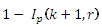# SQL Server NEGBINOM.DIST function

NEGBINOMDISTP

Updated: 31 July 2010

Use NEGBINOMDISTP to calculate the cumulative distribution function of the negative binomial distribution.

The formula for the cumulative distribution function is:Syntax
SELECT [wctStatistics].[wct].[NEGBINOMDISTP] (
<@Number_f, float,>
,<@Number_s, float,>
,<@Probability_s, float,>)

Arguments
@Number_f
is the number of failures. @Number_f is an expression of type float or of a type that implicitly converts to float
@Number_s
is the number of successes. @Number_s is an expression of type float or of a type that implicitly converts to float
@Probability_s
is the probability of success. @Probability_s is an expression of type float or of a type that implicitly converts to float
Return Types
float
Remarks
·         @Probability_s must be greater than or equal to zero and less than or equal to one (0 ≤ @Probability_s ≤ 1).
·         @Number_f   must be greater than or equal to zero (0 ≤ @Number_f).
·         @Number_s must be greater than or equal to one (1≤ @Number_s).
·         @Number_f is truncated to an integer.
·         @Number_s is truncated to an integer.
Examples
SELECT wct.NEGBINOMDISTP(4,90,0.975)
This produces the following result
----------------------
0.912840044254704

(1 row(s) affected)

You can use the SeriesInt function from the XLeratorDB/math library to generate a dataset which can be pasted into EXCEL to generate a graph of the cumulative distribution function.
SELECT SeriesValue
,wct.NEGBINOMDISTP(SeriesValue, 4, 0.4) as [f(x,4,0.4)]
,wct.NEGBINOMDISTP(SeriesValue, 4, 0.6) as [f(x,4,0.6)]
,wct.NEGBINOMDISTP(SeriesValue, 10, 0.4) as [f(x,10,0.4)]
,wct.NEGBINOMDISTP(SeriesValue, 10, 0.6) as [f(x,10,0.6)]
FROM wctMath.wct.SeriesInt(0,25,NULL,NULL,NULL)
This is an EXCEL-generated graph of the results### SupportCopyright 2008-2023 Westclintech LLC         Privacy Policy        Terms of Service